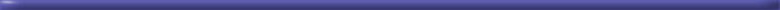Math TricksHere is a math trick so unbelievable that it will stump you. 1. Grab a calculator. (you won't be able to do this one in your head) 2. Key in the first three digits of your phone number (NOT the area code) 3. Multiply by 80 4. Add 1 5. Multiply by 250 6. Add the last 4 digits of your phone number 7. Add the last 4 digits of your phone number again. 8. Subtract 250 9. Divide number by 2 Do you recognize the answer?